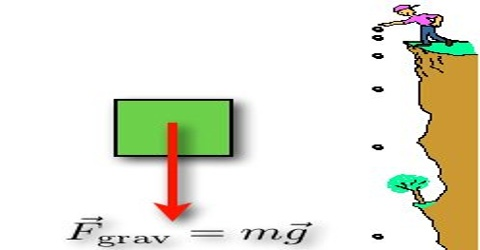Physics

# Force due to GravityForce due to gravity

An attractive force acts between any two bodies in this universe. Generally attraction between two bodies is called gravitation. But the attractive force between the earth and a body above or near the surface of the earth is called gravity. So, gravity is the special case of gravitation, i.e., gravity means gravitation of the earth.

If a body is allowed to fall, the body falls straight down due to gravity. The body also attracts the earth with equal and opposite force. Now the mass of the earth is many times heavier than anybody on the earth, hence any motion of the earth due to the attractive force of the body can be neglected. So, the body falls towards the earth. But the earth does not move towards the body.

If the earth is considered as a sphere of radius R we can consider that all its mass is concentrated at the centre of the earth. So, if the earth attracts a body on the surface of the earth of mass ‘m’ towards its centre with a force F, then according to Newton’s Law of gravitation.

F = [GMm/R2]

This very force towards the centre of the earth is the force due to gravity. So, the falling body actually approaches towards the centre of the earth due to gravity. For this reason, the suspended needle of the mason always points towards the centre of the earth. To bring down a body through the lever, to raise a body upward by a crane and to slip through the polished surface by children in children park- all these are work done by gravity. The force that causes a ball you throw in the air to come down again. The force that causes a glass you drop to fall to the floor.